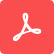# FDTD 101: Introduction to Finite- Difference Time-Domain Method

## Lecture 1: Introduction to FDTD Simulation

In this lecture, we will describe the basic concepts of EM simulation using FDTD.

- How Maxwell's equations can be solved computationally in the context of a photonic device.
- Discretization of the simulation space using the finite difference method.
- A demonstration on how we solve for the electromagnetic fields radiated by a dipole source.

For Python setup used in this lecture, please see related FDTD Python Tutorial below.